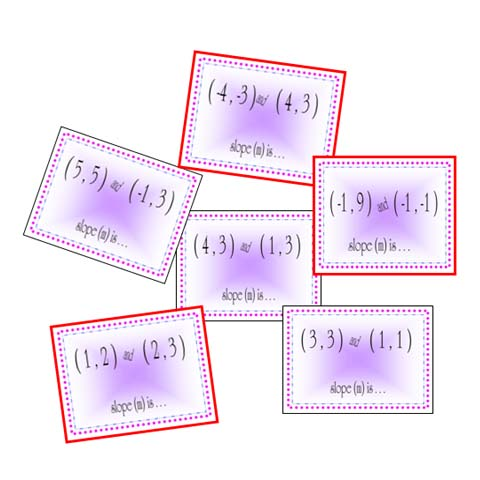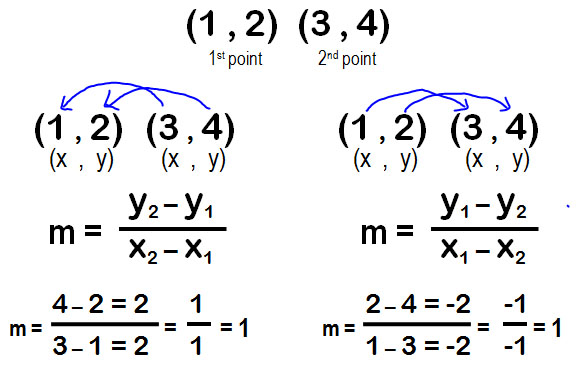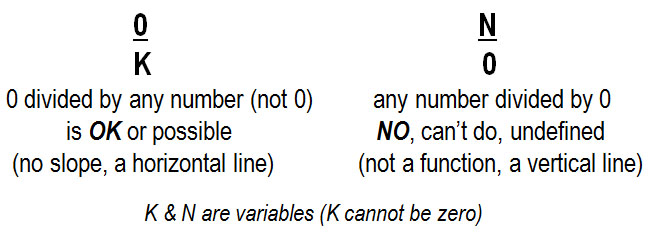STANDARD:  Algebra LEVELS:  M - MH   PURPOSE:  Apply rule for finding slope from 2 points.  (see tutorial for proof)           STRATEGY:  The rule says that slope can be found from 2 points by finding "the difference of the y's over the difference of the x's".  You must take the difference in the same order        either x & y in 1st point from x & y in 2nd point           or x & y in 2nd point from x & y in 1st pointFor example,The slope is 1/1 or -1/-1 or 1   Notice the answer is the same, whichever way you find the differences. Just do the same order between points. HINT: Decide which is the easiest!NO SLOPE:         points (4, 3) & (1, 3) become                                 3 - 3 over  1 - 4  =  0 / - 3                                slope (m) =  0 or no slope                            This is a horizontal line & the                                y coordinate is always  3                        (or can do as  3 - 3 over 4 - 1 =  0 / 3) UNDEFINED:    points  (-1, 9) & (-1, -1) become                              -1 - 9 over  -1 - (-1)  =  -10 / 0                                  Slope (m) = "undefined"                   Division by zero is considered undefined.                          This is a vertical line where every                           x will be -1; this is not a function.                 (or can do as 9 - (-1) over -1 - (-1) = 10 /0)A graphic organizer to remember 0 and division:DIRECTIONS:  Apply the rule using either rule and write the slope